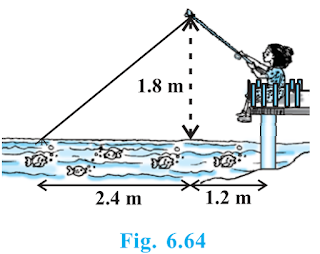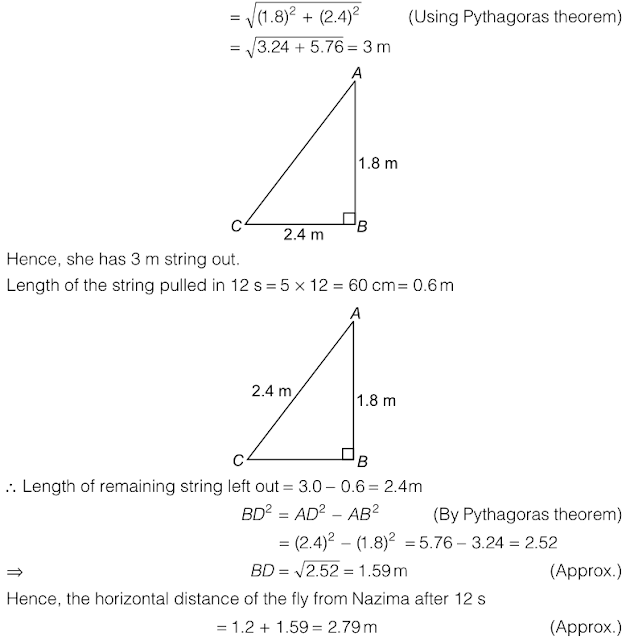# Class 10 Maths Chapter 6 Triangles

NCERT Solutions for Class 10 Maths Chapter 6 Triangles

Page No: 122

Exercise 6.1

1. Fill in the blanks using correct word given in the brackets:-

(i) All circles are __________. (congruent, similar)
► Similar

(ii) All squares are __________. (similar, congruent)
► Similar

(iii) All __________ triangles are similar. (isosceles, equilateral)
► Equilateral

(iv) Two polygons of the same number of sides are similar, if (a) their corresponding angles are __________ and (b) their corresponding sides are __________. (equal, proportional)
► (a) Equal, (b) Proportional

2. Give two different examples of pair of
(i) Similar figures
(ii) Non-similar figures

(i) Two twenty-rupee notes, Two two rupees coins.
(ii) One rupee coin and five rupees coin, One rupee not and ten rupees note.

3. State whether the following quadrilaterals are similar or not:The given two figures are not similar because their corresponding angles are not equal.

Page No: 128

Exercise 6.2

1. In figure.6.17. (i) and (ii), DE || BC. Find EC in (i) and AD in (ii).(i) In △ ABC, DE∥BC (Given)
∴ AD/DB = AE/EC [By using Basic proportionality theorem]
⇒ 1.5/3 = 1/EC
⇒ Σ EC = 3/1.5
EC = 3×10/15 = 2 cm
Hence, EC = 2 cm.

(ii) In △ ABC, DE∥BC (Given)
∴ AD/DB = AE/EC [By using Basic proportionality theorem]
⇒ AD = 1.8×7.2/5.4 = 18/10 × 72/10 × 10/54 = 24/10

2. E and F are points on the sides PQ and PR respectively of a ΔPQR. For each of the following cases, state whether EF || QR.
(i) PE = 3.9 cm, EQ = 3 cm, PF = 3.6 cm and FR = 2.4 cm
(ii) PE = 4 cm, QE = 4.5 cm, PF = 8 cm and RF = 9 cm
(iii) PQ = 1.28 cm, PR = 2.56 cm, PE = 0.18 cm and PF = 0.63 cmIn ΔPQR, E and F are two points on side PQ and PR respectively.
(i) PE = 3.9 cm, EQ = 3 cm (Given)
PF = 3.6 cm, FR = 2,4 cm (Given)
∴ PE/EQ = 3.9/3 = 39/30 = 13/10 = 1.3 [By using Basic proportionality theorem]
And, PF/FR = 3.6/2.4 = 36/24 = 3/2 = 1.5
So, PE/EQ ≠ PF/FR
Hence, EF is not parallel to QR.

(ii) PE = 4 cm, QE = 4.5 cm, PF = 8cm, RF = 9cm
∴ PE/QE = 4/4.5 = 40/45 = 8/9 [By using Basic proportionality theorem]
And, PF/RF = 8/9
So, PE/QE = PF/RF
Hence, EF is parallel to QR.

(iii) PQ = 1.28 cm, PR = 2.56 cm, PE = 0.18 cm, PF = 0.36 cm (Given)
Here, EQ = PQ - PE = 1.28 - 0.18 = 1.10 cm
And, FR = PR - PF = 2.56 - 0.36 = 2.20 cm
So, PE/EQ = 0.18/1.10 = 18/110 = 9/55 ... (i)
And, PE/FR = 0.36/2.20 = 36/220 = 9/55 ... (ii)
∴ PE/EQ = PF/FR.
Hence, EF is parallel to QR.

3. In the fig 6.18, if LM || CB and LN || CD, prove that AM/MB = AN/ADIn the given figure, LM || CB
By using basic proportionality theorem, we get,
AM/MB = AL/LC ... (i)
Similarly, LN || CD
∴ AN/AD = AL/LC ... (ii)
From (i) and (ii), we get

4. In the fig 6.19, DE||AC and DF||AE. Prove that
BF/FE = BE/EC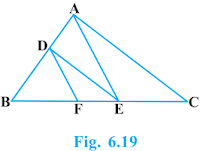In ΔABC, DE || AC (Given)
∴ BD/DA = BE/EC ...(i) [By using Basic Proportionality Theorem]

In  ΔABC, DF || AE (Given)
∴ BD/DA = BF/FE ...(ii) [By using Basic Proportionality Theorem]

From equation (i) and (ii), we get
BE/EC = BF/FE

5. In the fig 6.20, DE||OQ and DF||OR, show that EF||QR.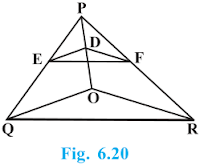In ΔPQO, DE || OQ (Given)
∴ PD/DO = PE/EQ ...(i) [By using Basic Proportionality Theorem]

In ΔPQO, DE || OQ (Given)
∴ PD/DO = PF/FR ...(ii) [By using Basic Proportionality Theorem]

From equation (i) and (ii), we get
PE/EQ = PF/FR

In ΔPQR, EF || QR. [By converse of Basic Proportionality Theorem]

6. In the fig 6.21, A, B and C are points on OP, OQ and OR respectively such that AB || PQ and AC || PR. Show that BC || QR.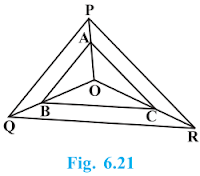In ΔOPQ, AB || PQ (Given)
∴ OA/AP = OB/BQ ...(i) [By using Basic Proportionality Theorem]

In ΔOPR, AC || PR (Given)
∴ OA/AP = OC/CR ...(ii) [By using Basic Proportionality Theorem]

From equation (i) and (ii), we get
OB/BQ = OC/CR

In ΔOQR, BC || QR. [By converse of Basic Proportionality Theorem].

7. Using Basic proportionality theorem, prove that a line drawn through the mid-points of one side of a triangle parallel to another side bisects the third side. (Recall that you have proved it in Class IX).Given: ΔABC in which D is the mid point of AB such that AD=DB.
A line parallel to BC intersects AC at E as shown in above figure such that DE || BC.

To Prove: E is the mid point of AC.

Proof: D is the mid-point of AB.
⇒ AD/BD = 1 ... (i)
In ΔABC, DE || BC,
Therefore, AD/DB = AE/EC [By using Basic Proportionality Theorem]

⇒1 = AE/EC [From equation (i)]
∴ AE =EC
Hence, E is the mid point of AC.

8. Using Converse of basic proportionality theorem, prove that the line joining the mid-points of any two sides of a triangle is parallel to the third side. (Recall that you have done it in Class IX).Given: ΔABC in which D and E are the mid points of AB and AC respectively such that AD=BD and AE=EC.

To Prove: DE || BC

Proof: D is the mid point of AB (Given)
⇒ AD/BD = 1 ... (i)

Also, E is the mid-point of AC (Given)
∴ AE=EC
⇒AE/EC = 1 [From equation (i)]

From equation (i) and (ii), we get
Hence, DE || BC [By converse of Basic Proportionality Theorem]

9. ABCD is a trapezium in which AB || DC and its diagonals intersect each other at the point O. Show that AO/BO = CO/DO.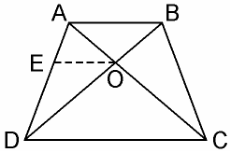Given: ABCD is a trapezium in which AB || DC in which diagonals AC and BD intersect each other at O.

To Prove: AO/BO = CO/DO

Construction: Through O, draw EO || DC || AB

OE || DC (By Construction)

∴ AE/ED = AO/CO  ...(i) [By using Basic Proportionality Theorem]

In ΔABD, we have
OE || AB (By Construction)

∴ DE/EA = DO/BO ...(ii) [By using Basic Proportionality Theorem]

From equation (i) and (ii), we get
AO/CO = BO/DO
⇒  AO/BO = CO/DO

10. The diagonals of a quadrilateral ABCD intersect each other at the point O such that AO/BO = CO/DO. Show that ABCD is a trapezium.Given: Quadrilateral ABCD in which diagonals AC and BD intersects each other at O such that AO/BO = CO/DO.

To Prove: ABCD is a trapezium

Construction: Through O, draw line EO, where EO || AB, which meets AD at E.

Proof: In ΔDAB, we have
EO || AB
∴ DE/EA = DO/OB ...(i) [By using Basic Proportionality Theorem]
Also,  AO/BO = CO/DO (Given)
⇒ AO/CO = BO/DO
⇒ CO/AO = BO/DO
⇒ DO/OB = CO/AO ...(ii)

From equation (i) and (ii), we get
DE/EA = CO/AO
Therefore, By using converse of Basic Proportionality Theorem, EO || DC also EO || AB
⇒ AB || DC.
Hence, quadrilateral ABCD is a trapezium with AB || CD.

Page No: 138

Exercise 6.3

1. State which pairs of triangles in Fig. 6.34 are similar. Write the similarity criterion used by you for answering the question and also write the pairs of similar triangles in the symbolic form:(i) In  ΔABC and ΔPQR, we have
∠A = ∠P = 60° (Given)
∠B = ∠Q = 80° (Given)
∠C = ∠R = 40° (Given)
∴ ΔABC ~ ΔPQR (AAA similarity criterion)

(ii) In  ΔABC and ΔPQR, we have
AB/QR = BC/RP = CA/PQ
∴  ΔABC ~ ΔQRP (SSS similarity criterion)

(iii) In ΔLMP and ΔDEF, we have
LM = 2.7, MP = 2, LP = 3, EF = 5, DE = 4, DF = 6
MP/DE = 2/4 = 1/2
PL/DF = 3/6 = 1/2
LM/EF= 2.7/5 = 27/50
Here, MP/DE = PL/DF ≠ LM/EF
Hence, ΔLMP and ΔDEF are not similar.

(iv) In ΔMNL and ΔQPR, we have
MN/QP = ML/QR = 1/2
∠M = ∠Q = 70°
∴ ΔMNL ~ ΔQPR (SAS similarity criterion)

(v) In ΔABC and ΔDEF, we have
AB = 2.5, BC = 3, ∠A = 80°, EF = 6, DF = 5, ∠F = 80°
Here, AB/DF = 2.5/5 = 1/2
And, BC/EF = 3/6 = 1/2
⇒ ∠B ≠ ∠F
Hence, ΔABC and ΔDEF are not similar.

(vi) In ΔDEF,we have
∠D + ∠E + ∠F = 180° (sum of angles of a triangle)
⇒ 70° + 80° + ∠F = 180°
⇒ ∠F = 180° - 70° - 80°
⇒ ∠F = 30°
In PQR, we have
∠P + ∠Q + ∠R = 180 (Sum of angles of Δ)
⇒ ∠P + 80° + 30° = 180°
⇒ ∠P = 180° - 80° -30°
⇒ ∠P = 70°
In ΔDEF and ΔPQR, we have
∠D = ∠P = 70°
∠F = ∠Q = 80°
∠F = ∠R = 30°
Hence, ΔDEF ~ ΔPQR (AAA similarity criterion)

Page No: 139

2.  In the fig 6.35, ΔODC ∝ ¼ ΔOBA, ∠ BOC = 125° and ∠ CDO = 70°. Find ∠ DOC, ∠ DCO and ∠ OAB.DOB is a straight line.
Therefore, ∠DOC + ∠ COB = 180°
⇒ ∠DOC = 180° - 125°
= 55°

In ΔDOC,
∠DCO + ∠ CDO + ∠ DOC = 180°
(Sum of the measures of the angles of a triangle is 180º.)
⇒ ∠DCO + 70º + 55º = 180°
⇒ ∠DCO = 55°
It is given that ΔODC ~ ΔOBA.
∴ ∠OAB = ∠OCD [Corresponding angles are equal in similar triangles.]
⇒ ∠ OAB = 55°
∴ ∠OAB = ∠OCD [Corresponding angles are equal in similar triangles.]
⇒ ∠OAB = 55°

3. Diagonals AC and BD of a trapezium ABCD with AB || DC intersect each other at the point O. Using a similarity criterion for two triangles, show that AO/OC = OB/ODIn ΔDOC and ΔBOA,
∠CDO = ∠ABO [Alternate interior angles as AB || CD]
∠DCO = ∠BAO [Alternate interior angles as AB || CD]
∠DOC = ∠BOA [Vertically opposite angles]
∴ ΔDOC ~ ΔBOA [AAA similarity criterion]
∴ DO/BO = OC/OA  [ Corresponding sides are proportional]
⇒ OA/OC = OB/OD

Page No: 140

4. In the fig.6.36, QR/QS = QT/PR and ∠1 = ∠2. Show that ΔPQS ~ ΔTQR.In ΔPQR, ∠PQR = ∠PRQ
∴ PQ = PR ...(i)
Given,QR/QS = QT/PR
Using (i), we get
QR/QS = QT/QP ...(ii)
In ΔPQS and ΔTQR,
QR/QS = QT/QP [using (ii)]
∠Q = ∠Q
∴ ΔPQS ~ ΔTQR [SAS similarity criterion]

5. S and T are point on sides PR and QR of ΔPQR such that ∠P = ∠RTS. Show that ΔRPQ ~ ΔRTS.In ΔRPQ and ΔRST,
∠RTS = ∠QPS (Given)
∠R = ∠R (Common angle)
∴ ΔRPQ ~ ΔRTS (By AA similarity criterion)

6. In the fig 6.37, if ΔABE ≅ ΔACD, show that ΔADE ~ ΔABC.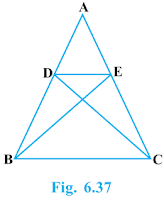It is given that ΔABE ≅ ΔACD.
∴ AB = AC [By cpct] ...(i)
And, AD = AE [By cpct] ...(ii)
AD/AB = AE/AC [Dividing equation (ii) by (i)]
∠A = ∠A [Common angle]
∴ ΔADE ~ ΔABC [By SAS similarity criterion]

7. In the fig 6.38, altitudes AD and CE of ΔABC intersect each other at the point P. Show that:(i) ΔAEP ~ ΔCDP
(ii) ΔABD ~ ΔCBE
(iv) ΔPDC ~ ΔBEC

(i) In ΔAEP and ΔCDP,
∠AEP = ∠CDP (Each 90°)
∠APE = ∠CPD (Vertically opposite angles)
Hence, by using AA similarity criterion,
ΔAEP ~ ΔCDP

(ii) In ΔABD and ΔCBE,
∠ABD = ∠CBE (Common)
Hence, by using AA similarity criterion,
ΔABD ~ ΔCBE

∠PAE = ∠DAB (Common)
Hence, by using AA similarity criterion,

(iv) In ΔPDC and ΔBEC,
∠PDC = ∠BEC (Each 90°)
∠PCD = ∠BCE (Common angle)
Hence, by using AA similarity criterion,
ΔPDC ~ ΔBEC

8. E is a point on the side AD produced of a parallelogram ABCD and BE intersects CD at F. Show that ΔABE ~ ΔCFB.In ΔABE and ΔCFB,
∠A = ∠C (Opposite angles of a parallelogram)
∠AEB = ∠CBF (Alternate interior angles as AE || BC)
∴ ΔABE ~ ΔCFB (By AA similarity criterion)

9. In the fig 6.39, ABC and AMP are two right triangles, right angled at B and M respectively, prove that:(i) ΔABC ~ ΔAMP
(ii) CA/PA = BC/MP

(i) In ΔABC and ΔAMP, we have
∠A = ∠A (common angle)
∠ABC = ∠AMP = 90° (each 90°)
∴  ΔABC ~ ΔAMP (By AA similarity criterion)

(ii) As, ΔABC ~ ΔAMP (By AA similarity criterion)
If two triangles are similar then the corresponding sides are equal,
Hence, CA/PA = BC/MP

10. CD and GH are respectively the bisectors of ∠ACB and ∠EGF such that D and H lie on sides AB and FE of ΔABC and ΔEFG respectively. If ΔABC ~ ΔFEG, Show that:
(i) CD/GH = AC/FG
(ii) ΔDCB ~ ΔHGE
(iii) ΔDCA ~ ΔHGF(i) It is given that ΔABC ~ ΔFEG.
∴ ∠A = ∠F, ∠B = ∠E, and ∠ACB = ∠FGE
∠ACB = ∠FGE
∴ ∠ACD = ∠FGH (Angle bisector)
And, ∠DCB = ∠HGE (Angle bisector)
In ΔACD and ΔFGH,
∠A = ∠F (Proved above)
∠ACD = ∠FGH (Proved above)
∴ ΔACD ~ ΔFGH (By AA similarity criterion)
⇒ CD/GH = AC/FG

(ii) In ΔDCB and ΔHGE,
∠DCB = ∠HGE (Proved above)
∠B = ∠E (Proved above)
∴ ΔDCB ~ ΔHGE (By AA similarity criterion)
(iii) In ΔDCA and ΔHGF,
∠ACD = ∠FGH (Proved above)
∠A = ∠F (Proved above)
∴ ΔDCA ~ ΔHGF (By AA similarity criterion)

Page No: 141

11. In the following figure, E is a point on side CB produced of an isosceles triangle ABC with AB = AC. If AD ⊥ BC and EF ⊥ AC, prove that ΔABD ~ ΔECF.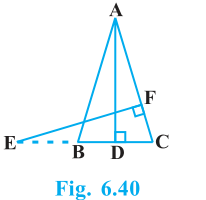It is given that ABC is an isosceles triangle.
∴ AB = AC
⇒ ∠ABD = ∠ECF
In ΔABD and ΔECF,
∴ ΔABD ~ ΔECF (By using AA similarity criterion)

12. Sides AB and BC and median AD of a triangle ABC are respectively proportional to sides PQ and QR and median PM of ΔPQR (see Fig 6.41). Show that ΔABC ~ ΔPQR.Given: ΔABC and ΔPQR, AB, BC and median AD of ΔABC are proportional to sides PQ, QR and median PM of ΔPQR
i.e., AB/PQ = BC/QR = AD/PM

To Prove: ΔABC ~ ΔPQR

Proof: AB/PQ = BC/QR = AD/PM
⇒ AB/PQ = BC/QR = AD/PM (D is the mid-point of BC. M is the mid point of QR)
⇒ ΔABD ~ ΔPQM [SSS similarity criterion]
∴ ∠ABD = ∠PQM [Corresponding angles of two similar triangles are equal]
⇒ ∠ABC = ∠PQR
In ΔABC and ΔPQR
AB/PQ = BC/QR ...(i)
∠ABC = ∠PQR ...(ii)
From equation (i) and (ii), we get
ΔABC ~ ΔPQR [By SAS similarity criterion]

13. D is a point on the side BC of a triangle ABC such that ∠ADC = ∠BAC. Show that CA2 = CB.CD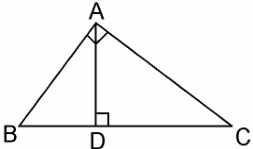∠ACD = ∠BCA (Common angle)
∴ ΔADC ~ ΔBAC (By AA similarity criterion)
We know that corresponding sides of similar triangles are in proportion.
∴ CA/CB =CD/CA
⇒ CA2 = CB.CD.

14. Sides AB and AC and median AD of a triangle ABC are respectively proportional to sides PQ and PR and median PM of another triangle PQR. Show that ΔABC ~ ΔPQR.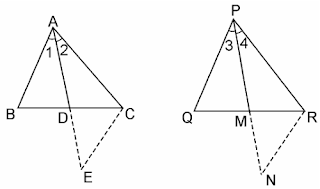Given: Two triangles ΔABC and ΔPQR in which AD and PM are medians such that AB/PQ = AC/PR = AD/PM

To Prove: ΔABC ~ ΔPQR

Construction: Produce AD to E so that AD = DE. Join CE, Similarly produce PM to N such that PM = MN, also Join RN.

Proof: In ΔABD and ΔCDE, we have
BD = DC [∴ AP is the median]
and, ∠ADB = ∠CDE [Vertically opp. angles]
∴ ΔABD ≅ ΔCDE [By SAS criterion of congruence]
⇒ AB = CE [CPCT] ...(i)
Also, in ΔPQM and ΔMNR, we have
PM = MN [By Construction]
QM = MR [∴ PM is the median]
and, ∠PMQ = ∠NMR [Vertically opposite angles]
∴ ΔPQM = ΔMNR [By SAS criterion of congruence]
⇒ PQ = RN [CPCT] ...(ii)
Now, AB/PQ = AC/PR = AD/PM
⇒ CE/RN = AC/PR = AD/PM ...[From (i) and (ii)]
⇒ CE/RN = AC/PR = 2AD/2PM
⇒ CE/RN = AC/PR = AE/PN [∴ 2AD = AE and 2PM = PN]
∴ ΔACE ~ ΔPRN [By SSS similarity criterion]
Therefore, ∠2 = ∠4
Similarly, ∠1 = ∠3
∴ ∠1 + ∠2 = ∠3 + ∠4
⇒ ∠A = ∠P ...(iii)
Now, In ΔABC and ΔPQR, we have
AB/PQ = AC/PR (Given)
∠A = ∠P [From (iii)]
∴ ΔABC ~ ΔPQR [By SAS similarity criterion]

15. A vertical pole of a length 6 m casts a shadow 4m long on the ground and at the same time a tower casts a shadow 28 m long. Find the height of the tower.Length of the vertical pole = 6m (Given)
Shadow of the pole = 4 m (Given)
Let Height of tower = h m
Length of shadow of the tower = 28 m (Given)
In ΔABC and ΔDEF,
∠C = ∠E (angular elevation of sum)
∠B = ∠F = 90°
∴ ΔABC ~ ΔDEF (By AA similarity criterion)
∴ AB/DF = BC/EF (If two triangles are similar corresponding sides are proportional)
∴ 6/h = 4/28
⇒ h = 6×28/4
⇒ h = 6 × 7
⇒ = 42 m
Hence, the height of the tower is 42 m.

16. If AD and PM are medians of triangles ABC and PQR, respectively where ΔABC ~ ΔPQR prove that AB/PQ = AD/PM.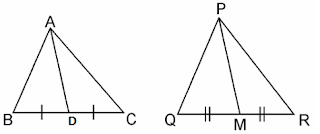It is given that ΔABC ~ ΔPQR
We know that the corresponding sides of similar triangles are in proportion.∴ AB/PQ = AC/PR = BC/QR ...(i)
Also, ∠A = ∠P, ∠B = ∠Q, ∠C = ∠R …(ii)
Since AD and PM are medians, they will divide their opposite sides.∴ BD = BC/2 and QM = QR/2 ...(iii)
From equations (i) and (iii), we get
AB/PQ = BD/QM ...(iv)
In ΔABD and ΔPQM,
∠B = ∠Q [Using equation (ii)]
AB/PQ = BD/QM [Using equation (iv)]
∴ ΔABD ~ ΔPQM (By SAS similarity criterion)⇒ AB/PQ = BD/QM = AD/PM.

Page No: 143

Exercise 6.4

1. Let ΔABC ~ ΔDEF and their areas be, respectively, 64 cm2 and 121 cm2. If EF = 15.4 cm, find BC.

It is given that,
Area of ΔABC = 64 cm2
Area of ΔDEF = 121 cm2
EF = 15.4 cm
and, ΔABC ~ ΔDEF
∴ Area of ΔABC/Area of ΔDEF = AB2/DE2
= AC2/DF2 = BC2/EF2 ...(i)
[If two triangles are similar, ratio of their areas are equal to the square of the ratio of their corresponding sides]
∴ 64/121 = BC2/EF2
⇒ (8/11)2 = (BC/15.4)2
⇒ 8/11 = BC/15.4
⇒ BC = 8×15.4/11
⇒ BC = 8 × 1.4
⇒ BC = 11.2 cm

2. Diagonals of a trapezium ABCD with AB || DC intersect each other at the point O. If AB = 2CD, find the ratio of the areas of triangles AOB and COD.ABCD is a trapezium with AB || DC. Diagonals AC and BD intersect each other at point O.
In ΔAOB and ΔCOD, we have
∠1 = ∠2 (Alternate angles)
∠3 = ∠4 (Alternate angles)
∠5 = ∠6 (Vertically opposite angle)
∴ ΔAOB ~ ΔCOD [By AAA similarity criterion]
Now, Area of (ΔAOB)/Area of (ΔCOD)
= AB2/CD2 [If two triangles are similar then the ratio of their areas are equal to the square of the ratio of their corresponding sides]
= (2CD)2/CD2 [∴ AB = CD]
∴ Area of (ΔAOB)/Area of (ΔCOD)
= 4CD2/CD = 4/1
Hence, the required ratio of the area of ΔAOB and ΔCOD = 4:1

3. In the fig 6.53, ABC and DBC are two triangles on the same base BC. If AD intersects BC at O, show that area (ΔABC)/area (ΔDBC) = AO/DO.Given: ABC and DBC are triangles on the same base BC. Ad intersects BC at O.

To Prove: area (ΔABC)/area (ΔDBC) = AO/DO.

Construction: Let us draw two perpendiculars AP and DM on line BC.

Proof: We know that area of a triangle = 1/2 × Base × Height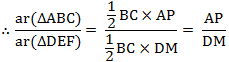In ΔAPO and ΔDMO,
∠APO = ∠DMO (Each equals to 90°)
∠AOP = ∠DOM (Vertically opposite angles)
∴ ΔAPO ~ ΔDMO (By AA similarity criterion)∴ AP/DM = AO/DO
⇒ area (ΔABC)/area (ΔDBC) = AO/DO.

4. If the areas of two similar triangles are equal, prove that they are congruent.Given: ΔABC and ΔPQR are similar and equal in area.

To Prove: ΔABC ≅ ΔPQR

Proof: Since, ΔABC ~ ΔPQR
∴ Area of (ΔABC)/Area of (ΔPQR) = BC2/QR2
⇒ BC2/QR2 =1 [Since, Area(ΔABC) = (ΔPQR)
⇒ BC2/QR2
⇒ BC = QR
Similarly, we can prove that
AB = PQ and AC = PR
Thus, ΔABC ≅ ΔPQR [BY SSS criterion of congruence]

5. D, E and F are respectively the mid-points of sides AB, BC and CA of ΔABC. Find the ratio of the area of ΔDEF and ΔABC.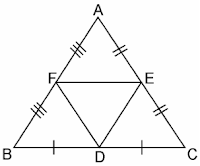Given: D, E and F are the mid-points of the sides AB, BC and CA respectively of the ΔABC.

To Find: area(ΔDEF) and area(ΔABC)

Solution: In ΔABC, we have
F is the mid point of AB (Given)
E is the mid-point of AC (Given)
So, by the mid-point theorem, we have
FE || BC and FE = 1/2BC
⇒ FE || BC and FE || BD [BD = 1/2BC]
∴ BDEF is parallelogram [Opposite sides of parallelogram are equal and parallel]
Similarly in ΔFBD and ΔDEF, we have
FB = DE (Opposite sides of parallelogram BDEF)
FD = FD (Common)
BD = FE (Opposite sides of parallelogram BDEF)
∴ ΔFBD ≅ ΔDEF
Similarly, we can prove that
ΔAFE ≅ ΔDEF
ΔEDC ≅ ΔDEF
If triangles are congruent,then they are equal in area.
So, area(ΔFBD) = area(ΔDEF) ...(i)
area(ΔAFE) = area(ΔDEF) ...(ii)
and, area(ΔEDC) = area(ΔDEF) ...(iii)
Now, area(ΔABC) = area(ΔFBD) + area(ΔDEF) + area(ΔAFE) + area(ΔEDC) ...(iv)
area(ΔABC) = area(ΔDEF) + area(ΔDEF) + area(ΔDEF) + area(ΔDEF)
⇒ area(ΔDEF) = 1/4area(ΔABC) [From (i)(ii) and (iii)]
⇒ area(ΔDEF)/area(ΔABC) = 1/4
Hence, area(ΔDEF):area(ΔABC) = 1:4

6. Prove that the ratio of the areas of two similar triangles is equal to the square of the ratio of their corresponding medians.Given: AM and DN are the medians of triangles ABC and DEF respectively and ΔABC ~ ΔDEF.

To Prove: area(ΔABC)/area(ΔDEF) = AM2/DN2

Proof: ΔABC ~ ΔDEF (Given)
∴ area(ΔABC)/area(ΔDEF) = (AB2/DE2) ...(i)
and, AB/DE = BC/EF = CA/FD ...(ii)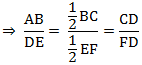In ΔABM and ΔDEN, we have
∠B = ∠E [Since ΔABC ~ ΔDEF]
AB/DE = BM/EN [Prove in (i)]
∴ ΔABC ~ ΔDEF [By SAS similarity criterion]
⇒ AB/DE = AM/DN ...(iii)
∴ ΔABM ~ ΔDEN
As the areas of two similar triangles are proportional to the squares of the corresponding sides.
∴ area(ΔABC)/area(ΔDEF) = AB2/DE2 = AM2/DN2

7. Prove that the area of an equilateral triangle described on one side of a square is equal to half the area of the equilateral triangle described on one of its diagonals.Given: ABCD is a square whose one diagonal is AC. ΔAPC and ΔBQC are two equilateral triangles described on the diagonals AC and side BC of the square ABCD.

To Prove: area(ΔBQC) = 1/2area(ΔAPC)

Proof: ΔAPC and ΔBQC are both equilateral triangles (Given)
∴ ΔAPC ~ ΔBQC [AAA similarity criterion]
∴ area(ΔAPC)/area(ΔBQC) = (AC2/BC2) = AC2/BC2⇒ area(ΔAPC) = 2 × area(ΔBQC)
⇒ area(ΔBQC) = 1/2area(ΔAPC)

Tick the correct answer and justify:

8. ABC and BDE are two equilateral triangles such that D is the mid-point of BC. Ratio of the area of triangles ABC and BDE is
(A) 2 : 1
(B) 1 : 2
(C) 4 : 1
(D) 1 : 4ΔABC and ΔBDE are two equilateral triangle. D is the mid point of BC.
∴ BD = DC = 1/2BC
Let each side of triangle is 2a.
As, ΔABC ~ ΔBDE
∴ area(ΔABC)/area(ΔBDE) = AB2/BD2 = (2a)2/(a)2 = 4a2/a2 = 4/1 = 4:1
Hence, the correct option is (C).

9. Sides of two similar triangles are in the ratio 4 : 9. Areas of these triangles are in the ratio
(A) 2 : 3
(B) 4 : 9
(C) 81 : 16
(D) 16 : 81Let ABC and DEF are two similarity triangles ΔABC ~ ΔDEF (Given)
and,  AB/DE = AC/DF = BC/EF = 4/9 (Given)
∴ area(ΔABC)/area(ΔDEF) = AB2/DE[the ratio of the areas of these triangles will be equal to the square of the ratio of the corresponding sides]
∴ area(ΔABC)/area(ΔDEF) = (4/9)= 16/81 = 16:81
Hence, the correct option is (D).

Page No: 150

Exercise 6.5

1.  Sides of triangles are given below. Determine which of them are right triangles? In case of a right triangle, write the length of its hypotenuse.
(i) 7 cm, 24 cm, 25 cm
(ii) 3 cm, 8 cm, 6 cm
(iii) 50 cm, 80 cm, 100 cm
(iv) 13 cm, 12 cm, 5 cm

(i) Given that the sides of the triangle are 7 cm, 24 cm, and 25 cm.
Squaring the lengths of these sides, we will get 49, 576, and 625.
49 + 576 = 625
(7)2 + (24)2 = (25)2
The sides of the given triangle are satisfying Pythagoras theorem.Hence, it is right angled triangle.
Length of Hypotenuse = 25 cm

(ii) Given that the sides of the triangle are 3 cm, 8 cm, and 6 cm.
Squaring the lengths of these sides, we will get 9, 64, and 36.
However, 9 + 36 ≠ 64
Or, 32 + 62 ≠ 82
Clearly, the sum of the squares of the lengths of two sides is not equal to the square of the length of the third side.
Therefore, the given triangle is not satisfying Pythagoras theorem.

(iii) Given that sides are 50 cm, 80 cm, and 100 cm.
Squaring the lengths of these sides, we will get 2500, 6400, and 10000.
However, 2500 + 6400 ≠ 10000
Or, 502 + 802 ≠ 1002
Clearly, the sum of the squares of the lengths of two sides is not equal to the square of the length of the third side.
Therefore, the given triangle is not satisfying Pythagoras theorem.
Hence, it is not a right triangle.

(iv) Given that sides are 13 cm, 12 cm, and 5 cm.
Squaring the lengths of these sides, we will get 169, 144, and 25.
Clearly, 144 +25 = 169
Or, 122 + 52 = 132
The sides of the given triangle are satisfying Pythagoras theorem.
Therefore, it is a right triangle.
Length of the hypotenuse of this triangle is 13 cm.

2. PQR is a triangle right angled at P and M is a point on QR such that PM ⊥ QR. Show that PM2 = QM × MR.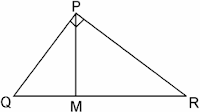Given: ΔPQR is right angled at P is a point on QR such that PM ⊥QR.

To prove: PM2 = QM × MR

Proof: In ΔPQM, we have
PQ2 = PM2 + QM2 [By Pythagoras theorem]
Or, PM2 = PQ2 - QM2 ...(i)
In ΔPMR, we have
PR2 = PM2 + MR2 [By Pythagoras theorem]
Or, PM2 = PR2 - MR2 ...(ii)
Adding (i) and (ii), we get
2PM2 = (PQ2 + PM2) - (QM2 + MR2)
= QR2 - QM2 - MR2        [∴ QR2 = PQ2 + PR2]
= (QM + MR)2 - QM2 - MR2
= 2QM × MR
∴ PM2 = QM × MR

3. In Fig. 6.53, ABD is a triangle right angled at A and AC ⊥ BD. Show that
(i) AB2 = BC × BD
(ii) AC2 = BC × DC
(iii) AD2 = BD × CD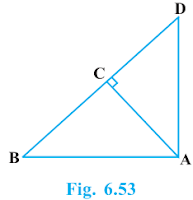(i) In ΔADB and ΔCAB, we have
∠DAB = ∠ACB (Each equals to 90°)
∠ABD = ∠CBA (Common angle)
∴ ΔADB ~ ΔCAB [AA similarity criterion]
⇒ AB/CB = BD/AB
⇒ AB2 = CB × BD

(ii) Let ∠CAB = x
In ΔCBA,
∠CBA = 180° - 90° - x
∠CBA = 90° - x
∠CAD = 90° - ∠CBA = 90° - x
∠CDA = 180° - 90° - (90° - x)
∠CDA = x
In ΔCBA and ΔCAD, we have
∠CAB = ∠CDA
∠ACB = ∠DCA (Each equals to 90°)
∴ ΔCBA ~ ΔCAD [By AAA similarity criterion]
⇒ AC/DC = BC/AC
⇒ AC2 =  DC × BC

(iii) In ΔDCA and ΔDAB, we have
∠DCA = ∠DAB (Each equals to 90°)
∴ ΔDCA ~ ΔDAB [By AA similarity criterion]
⇒ DC/DA = DA/DA
⇒ AD2 = BD × CD

4. ABC is an isosceles triangle right angled at C. Prove that AB2 = 2AC2 .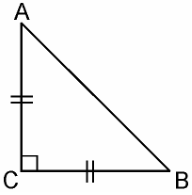Given that ΔABC is an isosceles triangle right angled at C.
In ΔACB, ∠C = 90°
AC = BC (Given)
AB2 = AC2 + BC2 ([By using Pythagoras theorem]
= AC2 + AC2 [Since, AC = BC]
AB2 = 2AC2

5. ABC is an isosceles triangle with AC = BC. If AB2 = 2AC2, prove that ABC is a right triangle.Given that ΔABC is an isosceles triangle having AC = BC and AB2 = 2AC2
In ΔACB,
AC = BC (Given)
AB2 = 2AC2 (Given)
AB2 = AC+ AC2
= AC2 + BC[Since, AC = BC]
Hence, By Pythagoras theorem ΔABC is right angle triangle.

6. ABC is an equilateral triangle of side 2a. Find each of its altitudes.ABC is an equilateral triangle of side 2a.
AB = AC [Given]
Hence, BD = DC [by CPCT]

7. Prove that the sum of the squares of the sides of rhombus is equal to the sum of the squares of its diagonals.ABCD is a rhombus whose diagonals AC and BD intersect at O. [Given]
We have to prove that,
AB+ BC+ CD2 + AD= AC+ BD2
Since, the diagonals of a rhombus bisect each other at right angles.
Therefore, AO = CO and BO = DO
In ΔAOB,
∠AOB = 90°
AB2 = AO+ BO... (i) [By Pythagoras]
Similarly,
DC2 = DO+ CO... (iii)
BC2 = CO+ BO... (iv)
Adding equations (i) + (ii) + (iii) + (iv)  we get,
AB+ AD+ DC+ BC2  =  2(AO+ BO+ DO+ CO)
= 4AO+ 4BO[Since, AO = CO and BO =DO]
= (2AO)2 + (2BO)2 = AC+ BD2

Page No: 151

8. In Fig. 6.54, O is a point in the interior of a triangleABC, OD ⊥ BC, OE ⊥ AC and OF ⊥ AB. Show that
(i) OA2 + OB2 + OC2 – OD2 – OE2 – OF2 = AF2 + BD2 + CE2 ,
(ii) AF2 + BD2 + CE2 = AE2 + CD2 + BF2.

Join OA, OB and OC(i) Applying Pythagoras theorem in ΔAOF, we have
OA2 = OF2 + AF2
Similarly, in ΔBOD
OB2 = OD2 + BD2
Similarly, in ΔCOE
OC2 = OE2 + EC2
OA2 + OB2 + OC2 = OF2 + AF2 + OD2 + BD2 + OE+ EC2
OA2 + OB2 + OC2 – OD2 – OE2 – OF2 = AF2 + BD2 + CE2.

(ii) AF2 + BD2 + EC2 = (OA2 - OE2) + (OC2 - OD2) + (OB2 - OF2)
∴ AF2 + BD2 + CE2 = AE2 + CD2 + BF2.

9. A ladder 10 m long reaches a window 8 m above the ground. Find the distance of the foot of the ladder from base of the wall.Let BA be the wall and Ac be the ladder,
Therefore, by Pythagoras theorem,we have
AC2 = AB2 + BC2
102 = 82 + BC2
BC= 100 - 64
BC= 36
BC = 6m
Therefore, the distance of the foot of the ladder from the base of the wall is 6 m.

10. A guy wire attached to a vertical pole of height 18 m is 24 m long and has a stake attached to the other end. How far from the base of the pole should the stake be driven so that the wire will be taut ?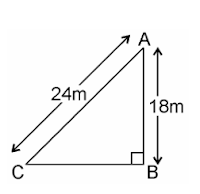Let AB be the pole and AC be the wire.
By Pythagoras theorem,
AC2 = AB2 + BC2
242 = 182 + BC2
BC= 576 - 324
BC= 252
BC = 6√7m
Therefore, the distance from the base is 6√7m.

11. An aeroplane leaves an airport and flies due north at a speed of 1,000 km per hour. At the same time, another aeroplane leaves the same airport and flies due west at a speed of 1,200 km per hour. How far apart will be the two planes afterhours?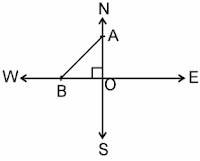Speed of first aeroplane = 1000 km/hr
Distance covered by first aeroplane due north in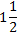hours (OA) = 100 × 3/2 km = 1500 km
Speed of second aeroplane = 1200 km/hr
Distance covered by second aeroplane due west inhours (OB) = 1200 × 3/2 km = 1800 km
In right angle ΔAOB, we have
AB2 = AO2 + OB2
⇒ AB2 = (1500)2 + (1800)2
⇒ AB = √2250000 + 3240000
= √5490000
⇒ AB = 300√61 km
Hence, the distance between two aeroplanes will be 300√61 km.

12. Two poles of heights 6 m and 11 m stand on a plane ground. If the distance between the feet of the poles is 12 m, find the distance between their tops.Let CD and AB be the poles of height 11 m and 6 m.
Therefore, CP = 11 - 6 = 5 m
From the figure, it can be observed that AP = 12m
Applying Pythagoras theorem for ΔAPC, we get
AP2 = PC2 + AC2
(12m)2 + (5m)2 = (AC)2
AC2 = (144+25)m2 = 169 m2
AC = 13m
Therefore, the distance between their tops is 13 m.

13. D and E are points on the sides CA and CB respectively of a triangle ABC right angled at C. Prove that AE2 + BD2 = AB2 + DE2.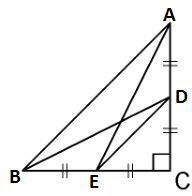Applying Pythagoras theorem in ΔACE, we get
AC2 + CE2 = AE2 ....(i)
Applying Pythagoras theorem in ΔBCD, we get
BC2 + CD2 = BD2 ....(ii)
Using equations (i) and (ii), we get
AC2 + CE2 + BC2 + CD2 = AE2 + BD2 ...(iii)
Applying Pythagoras theorem in ΔCDE, we get
DE2 = CD2 + CE2
Applying Pythagoras theorem in ΔABC, we get
AB2 = AC2 + CB2
Putting these values in equation (iii), we get
DE2 + AB2 = AE2 + BD2.

14. The perpendicular from A on side BC of a Δ ABC intersects BC at D such that DB = 3CD (see Fig. 6.55). Prove that 2AB2 = 2AC2 + BC2.Given that in ΔABC, we have
AD ⊥BC and BD = 3CD
AB2 = AD2 + BD2 ...(i)
AC2 = AD2 + DC2 ...(ii) [By Pythagoras theorem]
Subtracting equation (ii) from equation (i), we get
AB2 - AC2 = BD2 - DC2
= 9CD2 - CD2  [∴ BD = 3CD]
= 9CD2 = 8(BC/4)[Since, BC = DB + CD = 3CD + CD = 4CD]
Therefore, AB2 - AC2 = BC2/2
⇒ 2(AB2 - AC2) = BC2
⇒ 2AB2 - 2AC2 = BC2
∴ 2AB2 = 2AC2 + BC2.

15.  In an equilateral triangle ABC, D is a point on side BC such that BD = 1/3BC. Prove that 9AD2 = 7AB2.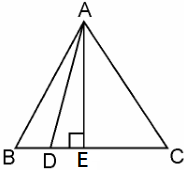Let the side of the equilateral triangle be a, and AE be the altitude of ΔABC.
∴ BE = EC = BC/2 = a/2
And, AE = a3/2
Given that, BD = 1/3BC
∴ BD = a/3
DE = BE - BD = a/2 - a/3 = a/6
Applying Pythagoras theorem in ΔADE, we get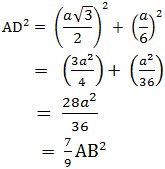⇒ 9 AD2 = 7 AB2

16. In an equilateral triangle, prove that three times the square of one side is equal to four times the square of one of its altitudes.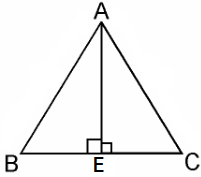Let the side of the equilateral triangle be a, and AE be the altitude of ΔABC.
∴ BE = EC = BC/2 = a/2
Applying Pythagoras theorem in ΔABE, we get
AB2 = AE2 + BE2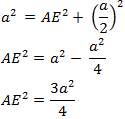4AE2 = 3a2
⇒ 4 × (Square of altitude) = 3 × (Square of one side)

17. Tick the correct answer and justify: In ΔABC, AB = 6√3 cm, AC = 12 cm and BC = 6 cm.
The angle B is:
(A) 120°
(B) 60°
(C) 90°
(D) 45°Given that, AB = 6√3 cm, AC = 12 cm, and BC = 6 cm
We can observe that
AB2 = 108
AC2 = 144
And, BC2 = 36
AB2 + BC2 = AC2
The given triangle, ΔABC, is satisfying Pythagoras theorem.
Therefore, the triangle is a right triangle, right-angled at B.
∴ ∠B = 90°
Hence, the correct option is (C).

Page No. 152

Exercise 6.6

1. In Fig. 6.56, PS is the bisector of ∠QPR of ΔPQR. Prove that QS/SR = PQ/PRGiven, in figure, PS is the bisector of ∠QPR of ∆PQR.
Now, draw RT SP || to meet QP produced in T.

Proof:

∵ RT SP || and transversal PR intersects them
∴ ∠1 = ∠2 (Alternate interior angle)…(i)
∴ RT SP || and transversalQT intersects them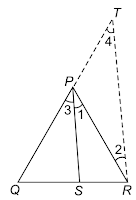∴ ∠3 = ∠4 (Corresponding angle) …(ii)
But ∠1 = ∠3 (Given)
∴ ∠2 = ∠4 [From Eqs. (i) and (ii)]
∴ PT = PR …(iii) (∵ Sides opposite to equal angles of a triangle are equal)
Now, in ∆QRT,
PS || RT (By construction)
∴ QS/SR = PQ/PT (By basic proportionally theorem)
⇒ QS/SR = PQ/PR [From Eq. (iii)]

2. In Fig. 6.57, D is a point on hypotenuse AC of ΔABC, such that BD ⊥ AC, DM ⊥ BC and
DN ⊥ AB. Prove that :
(i) DM2 = DN.MC
(ii) DN2 = DM.AN

Given that, D is a point on hypotenuse AC of ∆ABC, DM ⊥ BC and DN ⊥ AB.
Now, join NM.
Let BD and NM intersect at O.(i) In ∆DMC and ∆NDM,
∠DMC = ∠NDM (Each equal to 90°)
∠MCD = ∠DMN
Let MCD = ∠1
Then, ∠MDC = 90° − (90°-∠1)
= ∠1 (∵∠MCD + ∠MDC + ∠DMC = 180°)
∴ ∠ODM = 90° − (90° − ∠1)
= ∠1
⇒ ∠DMN = ∠1
∴ ∆DMO ~ ∆NDM  (AA similarity criterion)
∴ DM/ND = MC/DM
(Corresponding sides of the similar triangles are proportional)
⇒ DM2 = MC ND

∠NDM = ∠AND (Each equal to 90°)
Then, ∠NDA = 90° − ∠2
∵∠NDA + ∠DAN + ∠DNA = 180°
∴ ∠ODN = 90° − (90° − ∠2) = ∠2
∴ ∠DNO = ∠2
∴ ∆DNM ~ ∆NAD  (AA similarity criterion)
∴ DN/NA = DM/ND
⇒ DN/AN = DM/DN
⇒ DN2 = DM×AN

3. In Fig. 6.58, ABC is a triangle in which ∠ABC > 90° and AD ⊥ CB produced. Prove that AC2 = AB2 + BC2 + 2 BC.BD.Given that, in figure, ABC is a triangle in which ∠ABC> 90° and AD ⊥ CB produced.

Proof :

∠D = 90°
AC2 = AD2 + DC2  (By Pythagoras theorem)
= AD2 + (BD + BC)2 [∵DC = DB + BC]
= (AD2 + DB2) + BC2 + 2BD.BC [∵ (a + b)2 = a2 + b2 + 2ab]
= AB2 + BC2 + 2BC.BD

[∵In right ADB with ∠D = 90°, AB2 = AD2 + DB2] (By Pythagoras theorem)

4. In Fig. 6.59, ABC is a triangle in which ∠ABC < 90° and AD ⊥ BC. Prove that AC2 = AB2 + BC2 – 2BC.BD.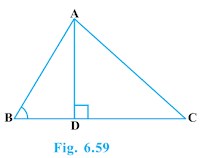Given that, in figure, ABC is a triangle in which ∠ABC < 90° and AD ⊥ BC.

Proof:

∠D = 90°
AC2 = AD2 + DC2  (By Pythagoras theorem)
= AD2 + (BC - BD)2  [∵BC = BD + DC]
= AD2 + (BC - BD)2  (BC = BD + DC)
= AD2 + BC2 + BD2 - 2BC.BD [∵ (a + b)2 = a2 + b2 + 2ab]
= (AD2 + BD2) + BC2 - 2BC . BD
= AB2 + BC2 - 2BC . BD
{In right △ADB with ∠D = 90°, AB2 = AD2 + BD2} (By Pythagoras theorem)

5. In Fig. 6.60, AD is a median of a triangle ABC and AM ⊥ BC. Prove that :
(i) AC2 = AD2 + BC.DM + (BC/2)2
(ii) AB2 = AD2 – BC.DM + (BC/2)2
(iii) AC2 + AB2 = 2 AD2 + 1/2 BC2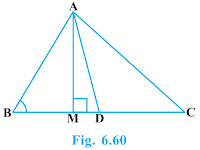Given that, in figure, AD is a median of a ∆ABC and AM ⊥ BC.
Proof:
(i) In right ∆AMC,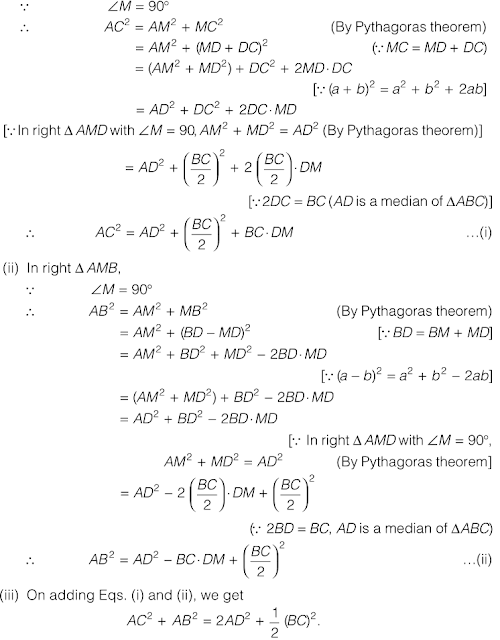6. Prove that the sum of the squares of the diagonals of parallelogram is equal to the sum of the squares of its sides.

Given that, ABCD is a parallelogram whose diagonals are AC and BD.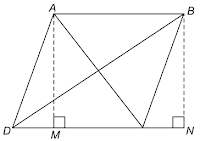Now, draw AM⊥DC and BN⊥D  (produced).
Proof:
In right ∆AMD and ∆BNC,
AD = BC (Opposite sides of a parallelogram)
AM = BN (Both are altitudes of the same parallelogram to the same base) ,
△AMD ⩭ △BNC (RHS congruence criterion)
MD = NC (CPCT) ---(i)

In right △BND,
∠N = 90°
BD2 = BN2 + DN2 (By Pythagoras theorem)
= BN2 + (DC + CN)2 (∵ DN = DC + CN)
= BN2 + DC2 + CN2 + 2DC.CN [∵ (a + b)2 = a2 + b2 + 2ab]
= (BN2 + CN2) + DC2 + 2DC.CN
= BC2 + DC2 + 2DC.CN --- (ii) (∵In right △BNC with ∠N = 90°)
BN2 + CN2 = BC2  (By Pythagoras theorem)

In right △AMC,
∠M = 90°
AC2 = AM2 + MC2 (∵MC = DC - DM)
= AM2 + (DC - DM)2 [∵ (a + b)2 = a2 + b2 + 2ab]
= AM2 + DC2 + DM2 - 2DC.DM
(AM2 + DM2) + DC2 - 2DC.DM
= AD2 + DC2 - 2DC.DM
[∵ In  right triangle AMD with ∠M = 90°, AD2 = AM2 + DM2 (By Pythagoras theorem)]
= AD2 + AB2 = 2DC.CN  --- (iii)
[∵ DC = AB, opposite sides of parallelogram and BM = CN from eq (i)]
Now, on adding Eqs. (iii) and (ii), we get
AC2 + BD2 = (AD2 + AB2) + (BC2 + DC2)
= AB2 + BC2 + CD2 + DA2

7. In Fig. 6.61, two chords AB and CD intersect each other at the point P. Prove that :
(i) Δ APC ~ Δ DPB
(ii) AP . PB = CP . DPGiven that, in figure, two chords AB and CD intersects each other at the point P.

Proof:

(i) ∆APC and ∆DPB
∠APC = ∠DPB (Vertically opposite angles)
∠CAP = ∠BDP (Angles in the same segment)
∴ ∆APC ~ ∆DPB (AA similarity criterion)

(ii) ∆APC ~ ∆DPB [Proved in (i)]
∴ AP/DP = CP/BP
(∴ Corresponding sides of two similar triangles are proportional)
⇒ AP.BP = CP.DP
⇒ AP.PB = CP.DP

8. In Fig. 6.62, two chords AB and CD of a circle intersect each other at the point P (when produced) outside the circle. Prove that
(i) Δ PAC ~ Δ PDB
(ii) PA.PB = PC.PD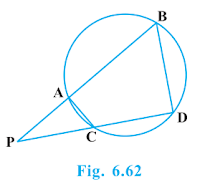Given that, in figure, two chords AB and CD of a circle intersect each other at the point P (when produced) out the circle.

Proof:

(i) We know that, in a cyclic quadrilaterals, the exterior angle is equal to the interior opposite angle.
Therefore, ∠PAC = ∠PDB …(i)
and ∠PCA = ∠PBD …(ii)
In view of Eqs. (i) and (ii), we get
∆PAC ~ ∆PDB  (∵ AA similarity criterion)

(ii) ∆PAC ~ ∆PDB  [Proved in (i)]
∴ PA/PD = PC/PB
(∵ Corresponding sides of the similar triangles are proportional)
⇒ PA.PB = PC.PD

9. In Fig. 6.63, D is a point on side BC of ΔABC such that BD/CD = AB/AC. Prove that AD is the bisector of ∠BAC.